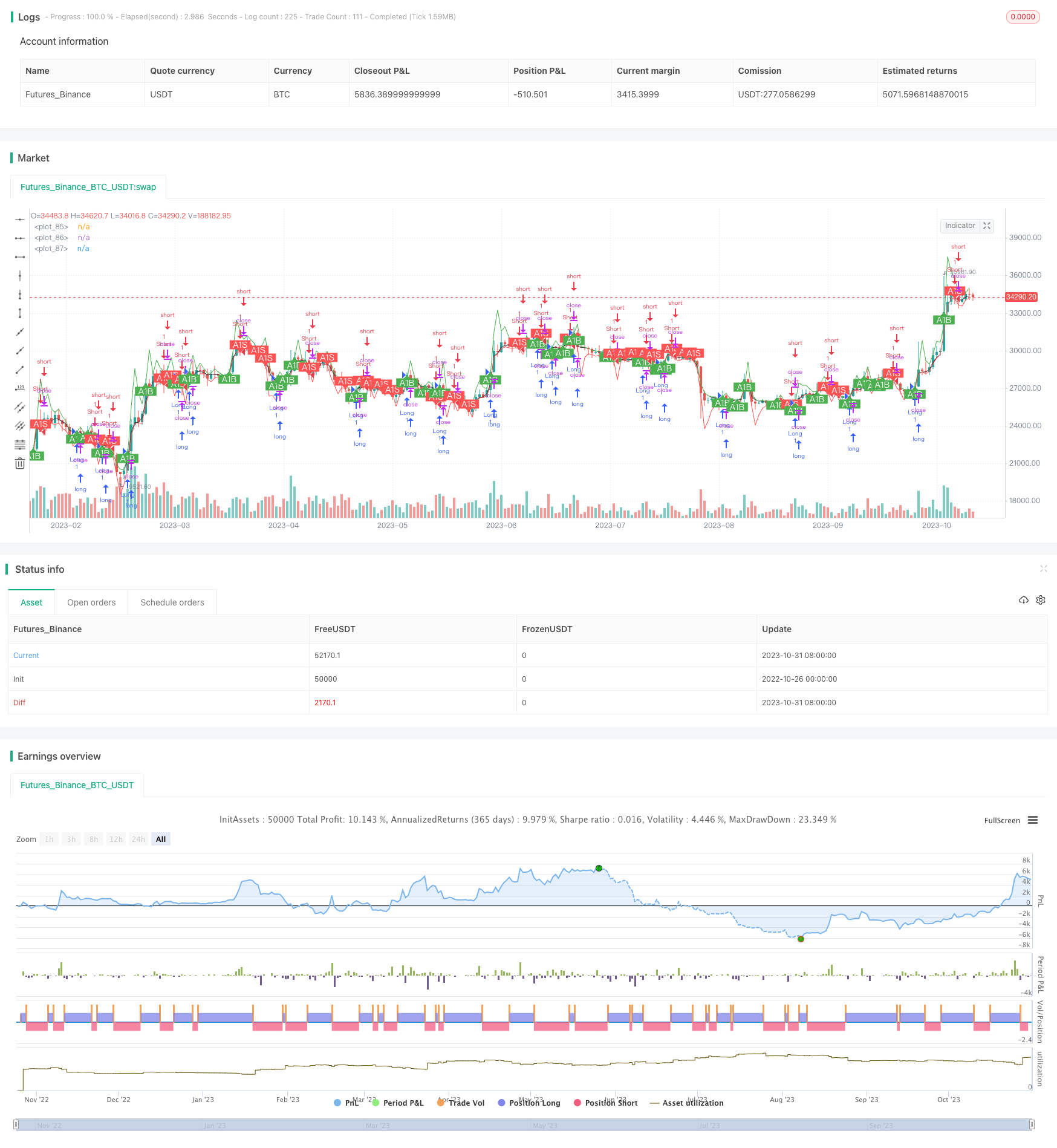# Adaptive Moving Average Channel Breakout Strategy

Author: ChaoZhang, Date: 2023-11-02 15:05:56
Tags:## Overview

The Adaptive Moving Average Channel Breakout Strategy is a trend-following breakout strategy based on the Adaptive Moving Average (AMA) and an adaptive channel range for generating trading signals. It uses the AMA to determine the current price trend direction and adaptive channel levels to detect price breakout signals for timely entries and exits.

## Strategy Principle

The core indicator of this strategy is the Adaptive Moving Average (AMA), which is used to capture the price trend. The AMA is calculated as:

AMA(t) = α(t-1) * P(t) + [1 - α(t-1)] * AMA(t-1)

Where P(t) is the current price, and α(t) is the smoothing constant between 0 and 1. α(t) is dynamically adjusted according to certain rules to control the sensitivity of the AMA to price changes. Specifically, α(t) is proportional to the deviation SNRT between AMA and price, which is calculated as:

SNRT = (P(t) - AMA(t-1)) / AMA(t-1)

Thus, as price fluctuations increase, α(t) will increase to make the AMA more responsive; when fluctuations decrease, α(t) will decrease to make the AMA smoother.

Based on the AMA, the strategy builds an adaptive channel range to detect price breakout signals. The upper and lower channel levels are:

Upper: H(t) = (1 + β*H(t-1)) * AMA(t)

Lower: L(t) = (1 - β*L(t-1)) * AMA(t)

Where β is an adjustable parameter controlling the channel width. Finally, the strategy generates trades based on price breakouts of the channel levels:

• Enter long when price breaks above the upper level.

• Enter short when price breaks below the lower level.

• Otherwise, stay flat.

The advantages of this strategy include:

1. The AMA is more flexible in capturing price trends compared to simple moving averages, especially in volatile markets.

2. The adaptive channel can dynamically adjust its width, expanding when the trend is unclear and narrowing when a trend emerges.

3. Price breakout signals can timely capture trend starts with higher win rates.

4. The logic is simple and clear, easy to understand and automate for quantitative trading.

## Risk Analysis

The risks of this strategy include:

1. Improper AMA parameters may miss price trends or generate false signals.

2. The adaptive channel parameters like β need careful tuning, otherwise too much whipsaw or missed trends may occur.

3. Price breakouts are susceptible to false breaks, requiring more filters.

4. The strategy does not incorporate risk management or stop loss mechanisms.

## Optimization Directions

The strategy can be optimized by:

1. Improving the α calculation to make the AMA more responsive.

2. Adding further confirmation after initial breakouts to avoid false signals.

3. Applying filters like volume or volatility to validate breakout validity.

4. Incorporating trailing stop loss to lock in profits and control risk.

5. Optimizing position sizing for different asset classes.

## Conclusion

In summary, the Adaptive Moving Average Channel Breakout Strategy is a simple and practical trend-following breakout strategy. It uses the flexible AMA to track price trends and an adaptive channel to detect breakout signals. The strategy has some advantages but also potential risks. With optimizations like parameter tuning, adding filters, and improving stops, it can become more robust. Overall, it provides a solid baseline model for quantitative trading.

```/*backtest
start: 2022-10-26 00:00:00
end: 2023-11-01 00:00:00
period: 1d
basePeriod: 1h
exchanges: [{"eid":"Futures_Binance","currency":"BTC_USDT"}]
*/

//@version=4

// CryptoStatistical - 2019
// AMA Strategy Channel Breakout Strategy from E. Durenard - Professional Automated Trading

strategy(title="[CS] AMA Strategy - Channel Break Out", shorttitle="AMA_ChannelBreakout_Strategy", initial_capital = 1000, overlay=true, pyramiding = 0, calc_on_every_tick=false, calc_on_order_fills=false, commission_type= strategy.commission.percent, commission_value = 0.08, currency=currency.USD)
testStartYear = input(2019, "Backtest Start Year")
testStartMonth = input(6, "Backtest Start Month")
testStartDay = input(1, "Backtest Start Day")
testPeriodStart = timestamp(testStartYear,testStartMonth,testStartDay,0,0)

testStopYear = input(2019, "Backtest Stop Year")
testStopMonth = input(12, "Backtest Stop Month")
testStopDay = input(31, "Backtest Stop Day")
testPeriodStop = timestamp(testStopYear,testStopMonth,testStopDay,0,0)

testPeriodBackground = input(title="Color Background?", type=input.bool, defval=true)
testPeriodBackgroundColor = testPeriodBackground and (time >= testPeriodStart) and (time <= testPeriodStop) ? #00FF00 : na
bgcolor(testPeriodBackgroundColor, transp=95)

testPeriod() =>  true

price = input(title='Price Source:', type=input.source, defval=close)

ama = price
hb = price
lb = price

// Static model parameters
minfactor = 0.
maxfactor = 1.
deviation_max = 1.
deviation_min = 1.
beta_hb = 1.
beta_lb = 1.
snr = 1.

normalized_atan= 0.
alpha = 0.5
// Suggested snr-factor from .5 upto 3.1 by .3 to find best parameter
snrfactor = input(title='SNR Factor:', type=input.float, minval=0.6, maxval=3.3, step=0.3, defval=2.1)

// Sensitivity Lookback search for the best perdiod from 5 to 20
lookback = input(title='Sensitivity Lookback:', type=input.integer, defval=5)

// Suggested Beta from .5 below 4.5 by .3, usually in the range 1.2, 1.5
beta = input(title='Beta:', type=input.float, minval=0.6, maxval=4.5, step=0.3, defval=2.1)

offsetlabel = input(title='Offset Label:', type=input.float, minval=0.001, maxval=0.03, step=0.001, defval=0.001)

// pi/2
pi2 = 1.5707963267948966

// Zero-lag resampled moving average (Durschner nwma)
f_nwma(_src, _period) =>
fast = _period/2
lambda = _period/fast
alpha = lambda * (_period - 1)/(_period - lambda)
average1 = wma(_src,_period)
average2 = wma(average1,fast)
nwma = (1+alpha)*average1 - alpha*average2

ama := alpha*price + (1-alpha)*nz(ama)

deviation_max := alpha*max((price - price)/price,0) + (1-alpha)*nz(deviation_max)
deviation_min := -alpha*min((price - price)/price,0) + (1-alpha)*nz(deviation_min)

beta_hb := beta*deviation_max
beta_lb := beta*deviation_min
hb := (1 + beta_hb)*ama
lb := (1 - beta_lb)*ama

snr := if price > hb
((price - ama)/ama)/beta_lb
else
if price < lb
-((price - ama)/ama)/beta_hb
else
0

normalized_atan := (atan(snrfactor*snr) + pi2)/(2*pi2)
alpha := f_nwma(minfactor + (maxfactor - minfactor)*normalized_atan, lookback)

plot(ama, color=color.black)
plot(hb, color=color.green)
plot(lb, color=color.red)

bc = false
// Sell Condition Var
sc = false
d = color.black

if(price > hb)
bc := true
d := color.green

// Sell Condition
if(price < lb)
sc := true
d := color.red

if(testPeriod())
strategy.entry("Long", strategy.long, when = bc)
strategy.entry("Short", strategy.short, when = sc)